Courses

# Boolean Algebra & Minimization Techniques - 2

## 20 Questions MCQ Test Topicwise Question Bank for Electrical Engineering | Boolean Algebra & Minimization Techniques - 2

Description
This mock test of Boolean Algebra & Minimization Techniques - 2 for Electrical Engineering (EE) helps you for every Electrical Engineering (EE) entrance exam. This contains 20 Multiple Choice Questions for Electrical Engineering (EE) Boolean Algebra & Minimization Techniques - 2 (mcq) to study with solutions a complete question bank. The solved questions answers in this Boolean Algebra & Minimization Techniques - 2 quiz give you a good mix of easy questions and tough questions. Electrical Engineering (EE) students definitely take this Boolean Algebra & Minimization Techniques - 2 exercise for a better result in the exam. You can find other Boolean Algebra & Minimization Techniques - 2 extra questions, long questions & short questions for Electrical Engineering (EE) on EduRev as well by searching above.
QUESTION: 1

### Consider the following statements associated with Boolean algebra: 1. There is nothing like subtraction or division in Boolean algebra. 2. There is possibility of having negative or fractional numbers. 3. A variable has no numerical significance. 4. Logical multiplication is same as the AND operation, and logical addition is the same as the OR operation. 5. There are only three constants within the Boolean system. Which of the statements given above are correct?

Solution:

Statement 2 and 5 are not correct because there is no possibility of having negative and fractional numbers is Boolean algebra. Also, there are only two constant 0 and 1 (don’t care (x) is not a constant) within the Boolean system.

QUESTION: 2

### Assertion (A): Boolean algebra and Binary number system are different from each other. Reason (R): There are some basic operations like AND, OR and NOT which are performed in Boolean algebra.

Solution:

Boolean algebra and Binary number system are different from each other because in Boolean algebra
1 + 1 = 1 while in the binary number system 1 + 1 = 10. There are lot of other examples to prove this.
Reason is also a correct statement because AND, OR and NOT are the three basic operations which are used in Boolean algebra. However, reason is not the correct explanation of assertion.

QUESTION: 3

### According to De Morgan’s theorem: 1. the NOR gate is equivalent to a bubbled NAND gate. 2. individual variables can be removed form under a NOT sign, and a sum of product from can be transformed to a product-of- sums form. 3. the complement of the product of variables is equal to the sum of their individual complements. 4. the NAND gate is equivalent to a bubbled NOR gate. 5. individual variables can be removed from under a NOT sign, and a product-of-sum form can be transformed to a sum-of-products form. Q. Which of the above statements about De Morgan’s theorem is/are not correct?

Solution:
•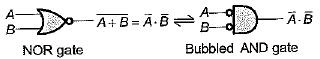Hence, statement-1 is not correct
•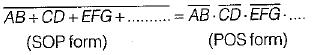Hence, statement-2 is correct.
•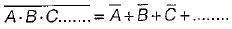Hence, staement-3 is correct.
•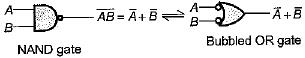Hence, statement-4 is not correct.
•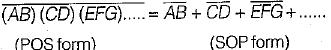Hence, statement 5 is correct.
QUESTION: 4

The complement of the expression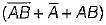is equal to

Solution: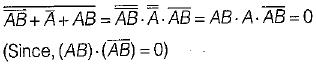QUESTION: 5

Match List-l (Boolean expressions)with List-ll (Minimized expression) and select the correct answer using the codes given below the lists:
List-l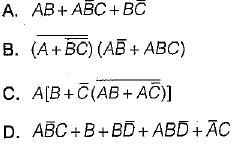List-II
1. 0
2. B + C
3. AC +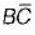4. AB
Codes:
A B C D
(a) 3 4 1 2
(b) 1 2 3 4
(c) 3 1 4 2
(d) 2 3 4 1

Solution:
•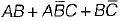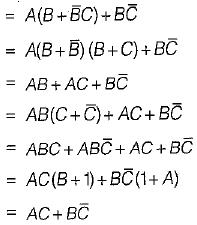•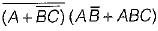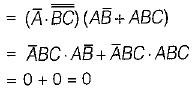•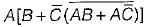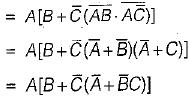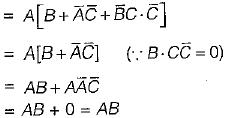•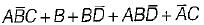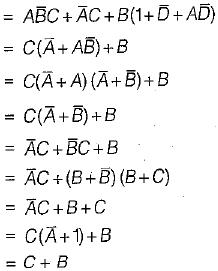QUESTION: 6

The minimized Boolean expression for the logic diagram shown below is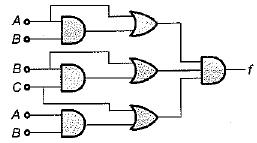Solution:

From given logic diagram,
f = (A+AB) (B + BC) (C + AB)
= A (1 + B).B(1 + C) (C + AB)
= AB (C + AB)
= ABC + AB.AB
= ABC + AB
= AB (C+1) = AB

QUESTION: 7

Assertion (A): The use of K-maps is usually limited to six-variables.
Reason (R): A K-map can not be used for problems involving more than six variables.

Solution:

A K-map map can be used for problems involving any number of variables. But, for more than six variables it becomes tedious to solve the problem sign K-map. Hence, the use oi K-map is usually limited to six-variables. Thus, reason is a false statement.

QUESTION: 8

Assertion (A): The binary number designations of the rows and columns of the K-map are in Gray code.
Reason (R): The Gray code designation is used in K-map to ensure that two physically adjacent squares are really adjacent i.e. their minterms or maxterms differ by only one variable.

Solution:
QUESTION: 9

Consider the following statements:
1. The terms which cannot be combined, further in the tabular method are called “prime implicants.’’
2. The implicants which will definitely occur in the final expression are called “essential prime implicants.”
3. The prime implicant chart is a pictorial representation of the relationships between the prime implicants and the minterms of the expression.
Which of the above statements is/are correct?

Solution:
QUESTION: 10

X8X4X2Xis an 8421 BCD inputto a logic circuit whose output is 1 when X8 = 0, X4 = 0 and X2 = 1, or when X8 = 0 and X4 = 1. The simplest output of this logic circuit would be:

Solution:

Denoting the non-complemented variable by 1 and the complemented variable by 0, the equation for the output is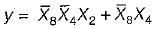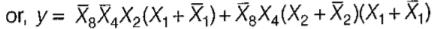= 0011 + 0010 + 0100 + 0101 + 0110 + 0111
= ∑M (2, 3, 4, 5, 6, 7)
The input is a 4-bit BCD, therefore there will be 6 invalid combinations (10,11,1.2,13,14,15) and the corresponding outputs are don’t cares.
Hence, the Boolean equation will be
y = ∑m ( 2, 3, 4, 5, 6, 7) + d(10, 11, 12, 13, 14, 15)
The K-map for the above Boolean equation is shown below: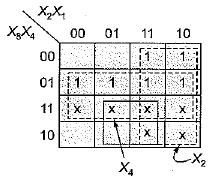From the above K-map, the simplex output of the given logic circuit will be: y = X2 + X4

QUESTION: 11

The inputs to a computer circuit are 4-bits of the binary number A3A2A1A0. The circuit is required to produce 1 if and only if ail of the following conditions hold.
1. The MSB is 1 or any of the other bits are 0.
2. A2 is 1 or any of the other bits are 0.
3. Any of the 4-bits are 0.
The minimized expression for the output of the computer circuit is

Solution:

From the given statements, the Boolean expression must be in the POS form given by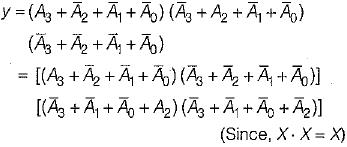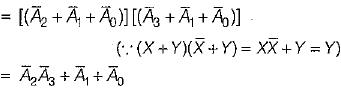QUESTION: 12

The minimum number of gate inputs required to implement the minimized output of the expression given by f are:
f = ∑m (1 ,5 , 6 , 12 , 13 , 14) + d(2, 4)

Solution:

Given, f = ∑m (1 ,5 ,6 ,12 ,13 ,14) + d(2 ,4) (In SOP form)
= πM (0, 3, 7, 8, 9, 10, 11, 15)-d(2, 4) (In POS form)
The K-map for SOP and POS expressions of output are shown below.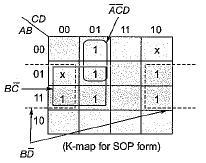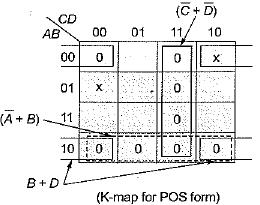In SOP form output is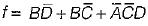In POS form output is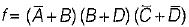The circuit realization for output fusing SOP and POS forms respectively are shown below: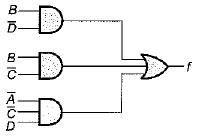(Using SOP output)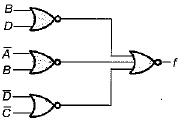(Using POS output)
We see that total number of gate inputs required using SOP output is 10 while that for POS output is 9. Hence, minimum number of inputs required to implement the minimized expression of output is 9 (using POS form).

QUESTION: 13

The minimized expression for the output given by: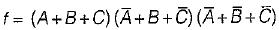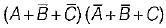Solution:

Given, f =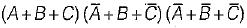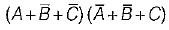The max terms are:
000, 101, 111, 011 ,110
= πM(0, 3, 5, 6, 7)
= ∑m(1, 2, 4)
The K-map is shown below.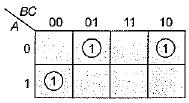Hence, output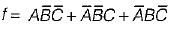QUESTION: 14

For 4 number of variables, maximum possible self dual expressions are

Solution:

For n number of variables, maximum possible self dual expressions are 22n-1
For n = 4, maximum self dual expressions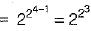= 28 = 256

QUESTION: 15

For the given Venn diagram, the minimized expression for the shaded area is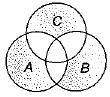Solution:

The shaded area represents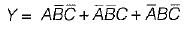QUESTION: 16

For the digital circuit shown below, the output is true if majority number of inputs are false.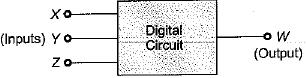The Boolean expression for the output is given by:

Solution:

The truth table for the input-output is shown below.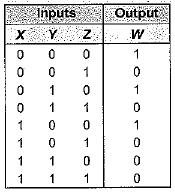Thus, output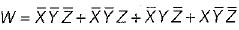QUESTION: 17

Match List-l (Logic circuit) with List-li (Output) and select the correct answer using the codes given below the lists:
List-I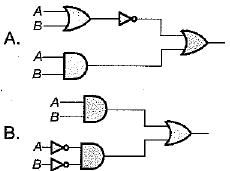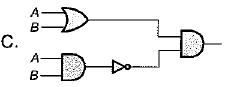List-II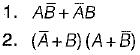3. AB
Codes:
A B C
(a) 3 3 2
(b) 1 2 1
(c) 2 1 3
(d) 2 2 1

Solution:

For logic circuit-A, output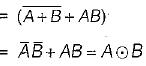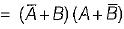For logic circuit-B, output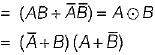For logic circuit-C, output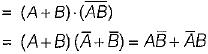QUESTION: 18

Match List-I (Boolean expression) with List-ll (Minimized expression) and select the correct answer using the codes given below the lists:
List-I
A. A. (A + B)
B. AB + BC +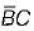C.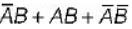D. A +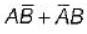List-II
1. A + B
2. AB + C
3. A
4.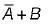Codes:
A B C D
(a) 3 2 4 1
(b) 2 4 3 1
(c) 1 2 3 4
(d) 3 4 1 2

Solution:
• A (A + B) = AA + AB = A + AB
= A(1 + B) = A
•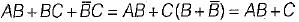•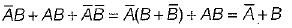•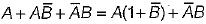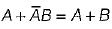QUESTION: 19

The minimized output equation of the K-map shown below has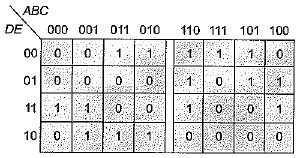Solution: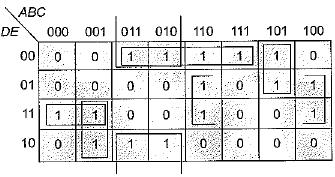From above K-map, output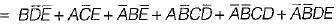Hence, number of minterms in the minimized output are 6.

QUESTION: 20

The simplified expression for the Boolean expression:
f(A, B, C, D) = ∑m (0, 2, 3, 6, 7) + ∑d (8,10,11,15)  is

Solution:

Given:
f = ∑m (0, 2, 3, 6, 7) + ∑d (8, 10, 11, 15)
= πM(1 , 4, 5, 9, 12, 13, 14) + πM(8, 10, 11, 15)
The K-map is shown below: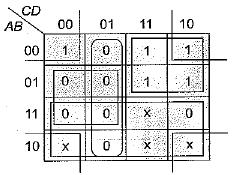Output in SOP form is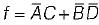.
Output in POS form is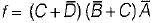Since output can be either in SOP form or in POS form, therefore option (c) is correct.